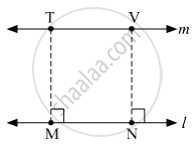SSC (English Medium) Class 8Maharashtra State Board
Share

# Draw a Line L. Take a Point T Outside the Line. Through Point T Draw a Line Parallel to Line L. - SSC (English Medium) Class 8 - Mathematics

ConceptTo Draw a Line Parallel to the Given Line

#### Question

Draw a line l. Take a point T outside the line. Through point T draw a line parallel to line l.

#### SolutionSteps of construction :
(1) Draw a line l. Take a point T outside the line l.
(2) Draw a segment MT ⊥ line l.
(3) Take another point N on line l.
(4) Draw a segment NV ⊥ line l, such that l(NV) = l(MT).
(5) Draw a line m passing through the points T and V.
Hence, the line m is the required line that passes through point T and parallel to line l.

Is there an error in this question or solution?

#### APPEARS IN

Balbharati Solution for Balbharati Class 8 Mathematics (2019 to Current)
Chapter 2: Parallel lines and transversals
Practice Set 2.3 | Q: 2 | Page no. 13
Solution Draw a Line L. Take a Point T Outside the Line. Through Point T Draw a Line Parallel to Line L. Concept: To Draw a Line Parallel to the Given Line.
S